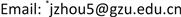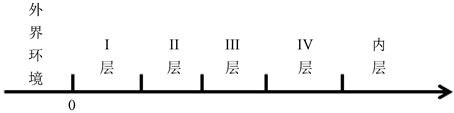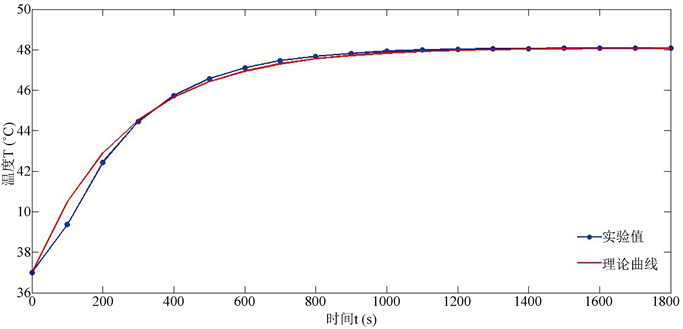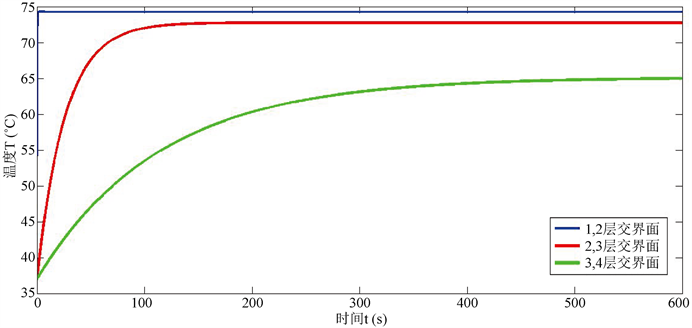﻿ 多层介质传热的计算模拟 Simulation of Heat Transfer in Multi-Layered Medium

Applied Physics
Vol. 09  No. 01 ( 2019 ), Article ID: 28414 , 6 pages
10.12677/APP.2019.91002

Simulation of Heat Transfer in Multi-Layered Medium

Jiapeng Zhen1, Qi Guo2, Jiang Zhou1*

1School of Physics, Guizhou University, Guiyang Guizhou

2School of Mathematics and Statistics, Guizhou University, Guiyang GuizhouReceived: Dec. 21st, 2018; accepted: Jan. 4th, 2019; published: Jan. 11th, 2019ABSTRACT

The temperature changes with time and location for heat transfer with steady heat source flowing through a multi-layered dielectric material. In this paper, the temperature distribution of steady heat source flowing through a multi-layered medium is studied. By using heat conduction equation, we derive the heat-stable temperature distribution and the temperature regularity of the innermost material changes as time. Our method is applicable to the composite problem of various thermal insulation materials, and can be used to determine the temperature distribution of the multi-layered medium material.

Keywords:Multi-Layered Media, Heat Transfer, Thermal Insulation Material

1贵州大学物理学院，贵州 贵阳

2贵州大学数学与统计学院，贵州 贵阳1. 引言

2. 模型的建立

2.1. 热传递温度分布Figure 1. The schematic of one dimensional multi-layered media

$\frac{\partial u\left(x,t\right)}{\partial t}-{a}^{2}\frac{{\partial }^{2}u\left(x,t\right)}{\partial {x}^{2}}=0$ , (1)

$u\left(x=0,t\right)={T}_{1}$ , $u\left(d,t=\infty \right)={T}_{5}$ . (2)

${X}_{k}\left(x\right)={p}_{k}\mathrm{cos}\left({\omega }_{k}x\right)+{q}_{k}\mathrm{sin}\left({\omega }_{k}x\right)$ , (3)

${T}_{k}\left(t\right)={n}_{k}{\text{e}}^{-{a}^{2}{\omega }_{k}^{2}t}$ ( $k=0,1,2,\cdots$ ) (4)

$u\left(x,t\right)=\underset{k}{\sum }{X}_{k}\left(x\right){T}_{k}\left(t\right)$ (5)

${Q}_{i}=A\frac{{\lambda }_{i}}{{d}_{i}}\left({T}_{i}-{T}_{i+1}\right)$ .

$\frac{\Delta {T}_{1,2}}{{R}_{1}}=\frac{\Delta {T}_{2,3}}{{R}_{2}}=\frac{\Delta {T}_{3,4}}{{R}_{3}}=\frac{\Delta {T}_{4,5}}{{R}_{4}}\equiv Q$ , (6)

2.2. 温度稳定前多层材料最内侧温度随时间的变化

$\begin{array}{l}\rho =\frac{{\rho }_{1}{v}_{1}+{\rho }_{2}{v}_{2}}{{v}_{1}+{v}_{2}},\\ c=\frac{{c}_{1}{v}_{1}+{c}_{2}{v}_{2}}{{v}_{1}+{v}_{2}},\\ \lambda =\frac{{\lambda }_{2}}{\left(\frac{{\lambda }_{2}}{{\lambda }_{1}}-1\right){v}_{1}+1}\end{array}$ (7)

$u\left(x=d,t\right)={C}_{1}{\text{e}}^{-{a}^{2}{\omega }^{2}t}+{C}_{0}$ , (8)

3. 计算模拟结果与讨论

$\rho =370.07,\text{\hspace{0.17em}}c=1622.68,\text{\hspace{0.17em}}\lambda =0.053,\text{\hspace{0.17em}}d=15.2$

$u\left(x=15.2,t\right)=-11.08{\text{e}}^{-0.0038t}+48.08$ .Figure 2. Comparison of fitted curve and experimental value

I、II层接触面：

$u\left(d=0.6,t\right)=-37.302{\text{e}}^{-5.4419t}+74.302$ .

II、III层接触面：

$u\left(d=6.6,t\right)=-35.754{\text{e}}^{-0.0385t}+72.754$ .

III、IV层接触面：

$u\left(d=10.2,t\right)=-28.117{\text{e}}^{-0.0088t}+65.117$ .Figure 3. The curve for temperature varies as time on three interfaces between layers

4. 实验探究

$\rho =1185.5,\text{\hspace{0.17em}}c=1210.1,\text{\hspace{0.17em}}\lambda =0.097,\text{\hspace{0.17em}}d=11.04$

$u\left(d=11.04,t\right)=-23.3{\text{e}}^{-0.0055t}+50$Figure 4. Comparison of fitted curve and experimental value

5. 总结

Simulation of Heat Transfer in Multi-Layered Medium[J]. 应用物理, 2019, 09(01): 7-12. https://doi.org/10.12677/APP.2019.91002

1. 1. 顾延安. 保温层外壁面温度的计算方法[J]. 化工设备设计, 1981(1): 1-4.

2. 2. 白净. 圆筒壁内的温度分布计算及分析[J]. 山西建筑, 2007, 33(25): 86-87.

3. 3. 曾剑, 刘云, 甄苇苇. 关于一类热传导方程的间断扩散系数的稳定方法[J]. 洛阳理工学院学报(自然科学版), 2017, 27(1): 80-83.

4. 4. 刘转转, 刘茂省, 张菊萍. 热传导方程的基本解与正态分布密度函数[J]. 西南民族大学学报(自然科学版), 2012, 38(2): 170-173.

5. 5. 苏新卫, 郭春晓. 热传导方程的解法注记[J]. 赤峰学院学报(自然科学版), 2018, 34(4): 11-12.

6. 6. 贾海峰, 刘蕤. 线性边界条件热传导方程求解[J]. 科教文汇, 2014(9): 47-50.

7. 7. 陈大伟, 等. 一维热传导过程的计算模拟[J]. 沈阳大学学报(自然科学版), 2018, 30(4): 338-341.

8. 8. 梁昆淼. 数学物理方法[M]. 北京: 高等教育出版社, 2010: 117-118.

9. 9. 陈则韶, 钱军. 复合材料等效导热系数的理论推算[J]. 中国科学技术大学学报, 1992, 22(4): 416-424.

NOTES

*通讯作者。